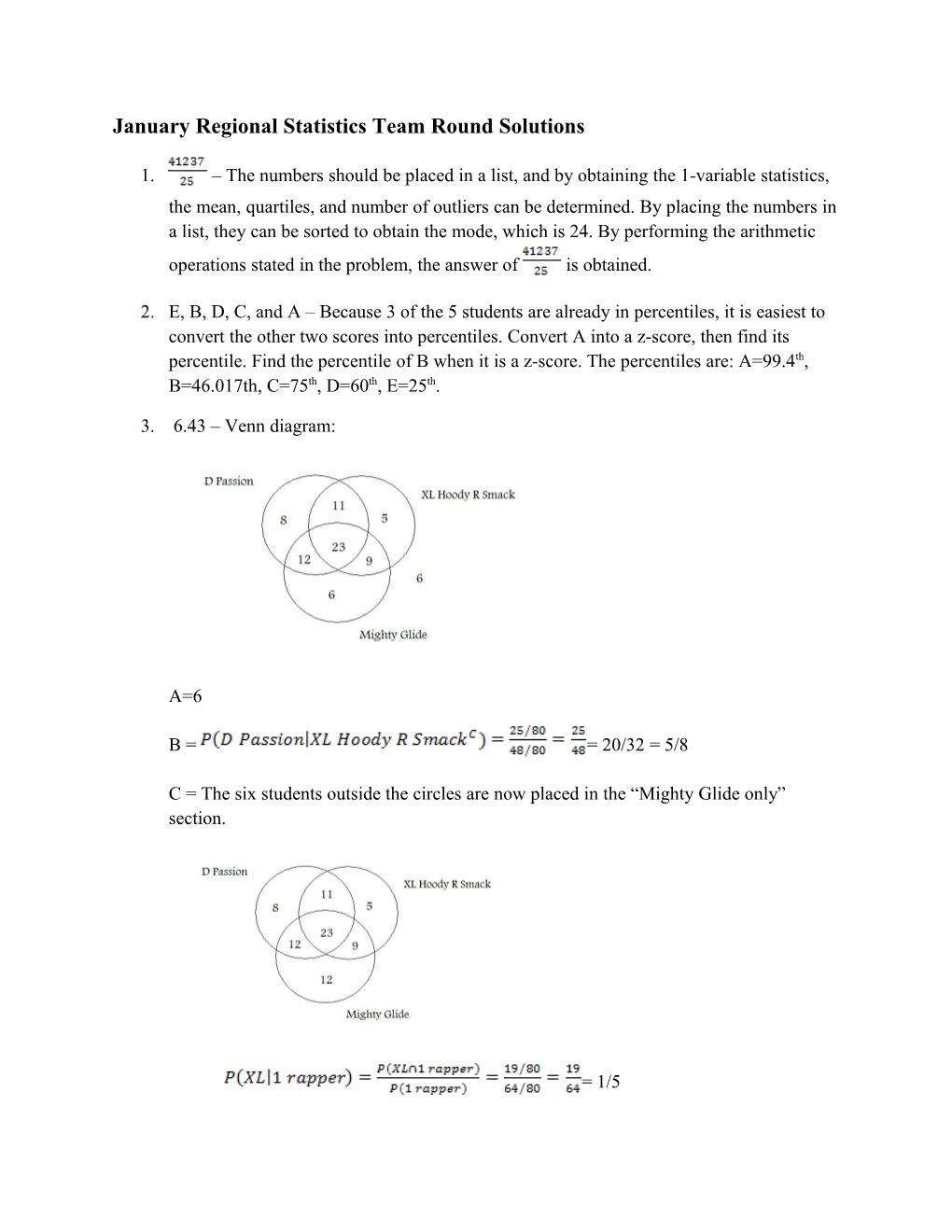# January Regional Statistics Team Round SolutionsJanuary Regional Statistics Team Round Solutions

1. – The numbers should be placed in a list, and by obtaining the 1-variable statistics, the mean, quartiles, and number of outliers can be determined. By placing the numbers in a list, they can be sorted to obtain the mode, which is 24. By performing the arithmetic operations stated in the problem, the answer of is obtained.
2. E, B, D, C, and A – Because 3 of the 5 students are already in percentiles, it is easiest to convert the other two scores into percentiles. Convert A into a z-score, then find its percentile. Find the percentile of B when it is a z-score. The percentiles are: A=99.4th, B=46.017th, C=75th, D=60th, E=25th.
3. 6.43 – Venn diagram:A=6

B = = 20/32 = 5/8

C = The six students outside the circles are now placed in the “Mighty Glide only” section.= 1/5

The sum gives you the answer: 6.43

1. 2.30575 – To create a line of best fit from the solutions:

and Line:

The coefficient of determination is r2. Dividing that by 5 gives you C, 0.1445.

January Regional Statistics Team Round Solutions

Plug the two x-values into the line of best fit, and do to obtain the residuals. Part D is 5.825.

1. –For A, the probability that they’re the same color is

B – You must find the probability of drawing a blue ball if a blue ball was placed in the urn and add it to the probability if ball was placed in the urn. The respective probabilities are .

C – Because one of the two balls placed is known to be blue, the two balls can either both be blue, or one is blue and one is gold.

The probability of both balls being blue is:

The probability of only one ball being blue is:

We multiply by 2 because the order that the balls are drawn: blue, then gold; gold, then blue.

We must find the probability that a blue ball drawn from the first urn was from the second urn, but split up into these two scenarios.

Both balls: One ball is blue:

The sum of both of these is the answer for C.

1. – All binompdf or binomcdf questions.

A = binompdf (10, .95, 10) = 0.60

B = binomcdf(10, .95, 8) = 0.09

C = 1 – binomcdf(10, .95, 7) = 0.99

D = binomcdf(10, .95, 7) – binomcdf(10, .95, 3) = 0.01

1. 345.626 – The first statement is true.

The second one is false, because the closer the coefficient is to 1, the stronger the correlation.

The third one is false, because it would not be possible to have the sample of the 2nd, 3rd, and 5th subjects with a sample size smaller than the population.

The fourth one is false, because the range is [-1,1].

The fifth one is true.

The sixth one is false, a statistic is used to describe a sample.

The seventh one is false, there is no set number of observations in a geometric distribution.

The eighth one is true, probabilities can only be obtained from ranges.

The ninth one is true.

1. 82.03 – A = 3(8) + 2(6) = 36

B =

C = 2(8) -5(6) = -14

January Regional Statistics Team Round Solutions

1. 1.72 – Using basic probability rules, such as , the probabilities for each part are found. The answers for each part are: A=, B=, C=, and D=.
2. : Part A is 33. Part B is 1. Part C is 14. The quadratic equation is x2+14x-33. is the positive root.
3. - The 68-95-99.7 empirical rule is used for this problem.

A = (-2σ, 2σ), which is 95%

B = (-σ, 3σ) which is .5(68%) + .5(99.7%) = 83.85%

C = (-∞, 2σ) and (3σ, ∞), which is .5(1-.95) + .5(1-.997) = 2.65%

D =

1. - The expected value of a geometric distribution is the reciprocal of the probability of success. A = 4.

B = geometpdf(.25,3) = 0.140625

C = At least 4 tries means 3 failures.

D = geometcdf(.25,5) – geometcdf(.25,2) = 0.3251953125

1. 191.7 – All of the answers can be found by putting the numbers into a list and finding the 1-variable statistics. The only calculation necessary is the IQR, which is Q3 – Q1.

A = 38.5

B = 64

C = 55

D = 34.2

1. The chi-square statistic is the sum of . The expected value for each of the fruits is 6. The statistic is 5. The degrees of freedom are n-1, which is 4 for this problem.